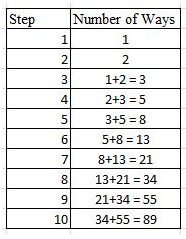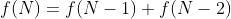MATHEMATICS / SECONDARY JUNIOR

# Climbing stairs and recurrence relations

3 OCTOBER 2021

Chapter 1: The Problem

Climbing up flights of stairs is an activity we do every day. How many steps can you climb up at one time? Most people can climb up two steps at a time; or maybe even three or four steps without losing their balance. How many ways can you climb up a flight of 10 stairs, if you can climb up to two steps at a time? It’s easy to write down a few ways that we can climb up the flight of steps: we can take 1 step ten times, or 2 steps five times, or 1 step twice and 2 steps four times…

The last case is different, as there are multiple ways to take 1 step twice and 2 steps four times. For example, we could climb to step

1, then 2, 4, 6, 8, 10; or climb to step 1, then 3, 5, 7, 9, 10. These two ways of climbing the steps are clearly distinct, as the steps taken are different in each case.

It would be extremely tedious to list all of the possible ways to climb the flight of stairs. Even if we list out the different cases and

solve each case separately with combinatorics, it would be time-consuming as there are many cases to consider. Is there a simpler approach to this problem?

Chapter 2: The Solution

When climbing up the flight of stairs, a pattern is clear: To reach a higher step, we must start from a lower step. Of course that is obvious, but how can we use that to solve the problem?

Let’s take a closer look at the first few steps. To get to step 1, there is only one way: We can take 1 step from the starting point. Let’s write this as 0+1, to represent taking 1 step from the starting point.

To get to step 2, there are two ways: We can take 1 step from step 1, or 1 step from step 2. This can be written as 0+2 or 0+1+1. To get to step 3, there are three ways: 0+1+2, 0+2+1, 0+1+1+1. To get to step 4, there are five ways: 0+1+2+1, 0+2+1+1, 0+1+1+1+1, 0+2+2, 0+1+1+2.

Do you notice a pattern? To get to any step, you can start

from two steps lower, and take two steps; or start from one step lower and take one step. Since we know that there is one way to get to step 1, and two ways to get to step 2, then: To get to step 3, we can either come from step 1 or step 2, thus we add the number of ways to get from step 1 and step 2, to get to step 3. Hence, there are 1+2 = 3 possible ways. To get to step 4, we can come from step 2 or step 3: 2+3 = 5 possible ways.

We can continue this pattern of addition all the way to step 10:Thus, there are 89 possible ways to climb to the top of the staircase.

Chapter 3: Representation

Is there a formula we can use for the number of ways to reach step N? If the flight of stairs had twenty steps instead, how can we find the number of ways to reach the top?

Let f(N) denote the number of ways to reach step N. f(N) is

a special type of function: it is not defined solely in terms of N, but in terms of f(N). As we have covered in the chapter above, to get to step N, we must have either come from step (N-1) or step (N-2), and the number of ways to reach step N is equal to the number of ways to reach step N-1, plus the number of ways to reach step N-2. Thus, we have reached the relationship below:This relationship is meaningless if we do not provide a

starting point. Fortunately, it is easy to work out the number of ways to reach the first and second step: f(1) = 1 and f(2) = 2.

With this formula, we can thus continue the pattern of addition to find out how to reach the 20th step, or even the 100th

step.

A recurrence function is a function that is defined as a

function of preceding terms of the same function. In this article, the recurrence relation described shows the relationship, which is the well-known Fibonacci sequence, where each term is equal to the sum of the two terms before itself.

Recurrence functions are prevalent in nature. In fact, the Fibonacci sequence was first used to describe the number of rabbits, if a pair of adult rabbits produces another pair of young rabbits every month, and young rabbits take one month to mature into adult rabbits. Other recurrence relations have been used to model the numbers of predator and prey in an ecosystem, or used to model the changes in price of different items in the economy.

1. One day before climbing the stairs, I noticed a spill on

the sixth step of the staircase. If I can climb up to 2 steps at a time, how many ways can I reach the top of the staircase (which has 10 steps) if I must avoid the sixth step?

2. After much stair-climbing, I can now climb up to 3 steps

at a time without losing my balance. How many ways are there to climb the same flight of 10 steps?

Author